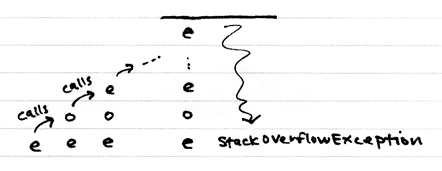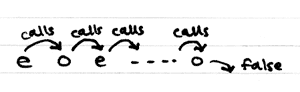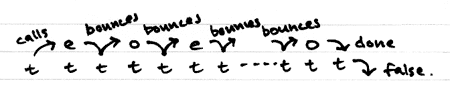# Tail calls, @tailrec and trampolines

Recursion is an essential part of functional programming. But if each call allocates a stack frame, then too much recursion will overflow the stack. Most functional programming languages solve this problem by eliminating stack frames through a process called tail-call optimisation. Unfortunately for Scala programmers, the JVM doesn't perform this optimisation.

Here's a picture of a Scala program as it executes. This program tries to work out whether 9999 is even or odd by calling `odd1` and `even1` recursively. The stack overflows before we can make 9999 calls.``````def odd1(n: Int): Boolean = {
if (n == 0) false
else even1(n - 1)
}
def even1(n: Int): Boolean = {
if (n == 0) true
else odd1(n - 1)
}
even1(9999)
``````

All the calls in our example program are in tail position, so if the JVM did support tail-call optimisation, then the program would be able to complete successfully.Luckily, even without JVM support, the Scala compiler can perform tail-call optimisation in some cases. The compiler looks for certain types of tail calls and translates them automatically into loops. At the moment it can optimise self calls in final methods and in local functions. It cannot optimise non-final methods (because they might be overridden by a subclass), and it cannot optimise tail calls that are made to different methods.

#### What this means

Because of these limitations, you need to be careful when using recursion in Scala. When writing programs, you will need to keep in mind how both the compiler and the JVM work. One safe approach is to use code from the standard library, where possible. For example, you'll find that many recursive algorithms can easily be rewritten in terms of standard operations like `map` and `fold`.

In Scala 2.8, you will also be able to use the new `@tailrec` annotation to get information about which methods are optimised. This annotation lets you mark specific methods that you hope the compiler will optimise. You will then get a warning if they are not optimised by the compiler. In Scala 2.7 or earlier, you will need to rely on manual testing, or inspection of the bytecode, to work out whether a method has been optimised.

If you do find a call that you think should be optimised by the compiler, but isn't, then you should check that the call:

1. is a tail call,
2. is in a final method or local function, and
3. is to itself.

For example, the code for `factorial` below would not be optimised. The call is not in tail position (the tail operation is the multiplication), and the method is public and non-final, so it could be overridden by a subclass.

``````class Factorial1 {
def factorial(n: Int): Int = {
if (n <= 1) 1
else n * factorial(n - 1)
}
}
``````

You can make simple changes to `factorial` to eliminate both of these problems. First, you could move the recursive code into a local function within the method, so that it cannot be overridden. Second, you could introduce an accumulator so that multiplication happens before the recursive call. Finally, you could add a `@tailrec` annotation so that you can be sure that your changes have worked.

``````import scala.annotation.tailrec

class Factorial2 {
def factorial(n: Int): Int = {
@tailrec def factorialAcc(acc: Int, n: Int): Int = {
if (n <= 1) acc
else factorialAcc(n * acc, n - 1)
}
factorialAcc(1, n)
}
}
``````

But there are some types of recursive code that the compiler will not be able to optimise. For example, if your code is mutually recursive, as it is with `odd1` and `even1`, then you will need to try something else. One thing you might consider, is using a trampoline.

#### Trampolines

A trampoline is a loop that repeatedly runs functions. Each function, called a thunk, returns the next function for the loop to run. The trampoline never runs more than one thunk at a time, so if you break up your program into small enough thunks and bounce each one off the trampoline, then you can be sure the stack won't grow too big.

Here is our program again, rewritten in trampolined style. `Call` objects contain the thunks and a `Done` object contains the final result. Instead of making a tail call directly, each method now returns its call as a thunk for the trampoline to run. This frees up the stack after each iteration. The effect is very similar to tail-call optimisation.``````def even2(n: Int): Bounce[Boolean] = {
if (n == 0) Done(true)
else Call(() => odd2(n - 1))
}
def odd2(n: Int): Bounce[Boolean] = {
if (n == 0) Done(false)
else Call(() => even2(n - 1))
}
trampoline(even2(9999))
``````

It only takes a few lines of code to implement a trampoline.

``````sealed trait Bounce[A]
case class Done[A](result: A) extends Bounce[A]
case class Call[A](thunk: () => Bounce[A]) extends Bounce[A]

def trampoline[A](bounce: Bounce[A]): A = bounce match {
case Call(thunk) => trampoline(thunk())
case Done(x) => x
}
``````

Trampolined code is harder to read and write, and it executes more slowly. However, trampolines can be invaluable when your program would otherwise run out of stack space, and the only other alternative is to convert it into an imperative style. There has recently been talk of including a trampoline implementation in Scala 2.8. (The code in this article is based on the code from that discussion.)

#### Postscript: Continuations

I've been writing about continuations quite a bit recently, so I think it's fitting to mention their relationship to trampolines. It turns out that a thunk can be easily manufactured from a continuation. You can create thunks automatically using `shift` and `reset`. In fact my recent implementation of `goto` used a form of trampoline. I'll close here by showing how `goto` can be implemented using the trampoline that we defined above.

``````import scala.continuations.cps
import scala.continuations.ControlContext.{shift,reset}

def trampolineK[A,B1<:C,C1<:C,C](body: => B1 @cps[B1,Bounce[C1]]): C =
trampoline(reset(Done(body)))

case class Label[A](k: Label[A] => Bounce[A])

def label[A]: Label[A] @cps[Bounce[A],Bounce[A]] =
shift((k: Label[A] => Bounce[A]) => k(Label(k)))

def goto[A](l: Label[A]): Unit @cps[Bounce[A],Bounce[A]] =
shift((k: Nothing => Bounce[A]) => Call(() => l.k(l)))

trampolineK {
var sum = 0
var i = 0
val beforeLoop = label
if (i < 10000) {
println(i)
sum += i
i += 1
goto(beforeLoop)
}
println(sum)
}
``````